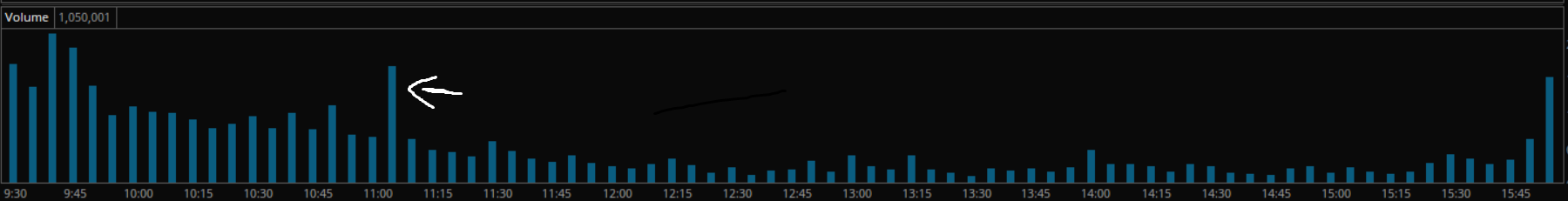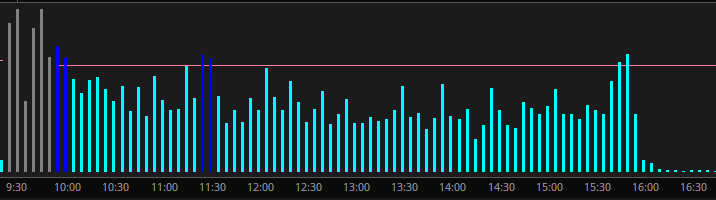#### bsp2710

##### New member
I would like to set up an alert for a specific type of volume spike. I think this is rather basic, however I have a limited knowledge of thinkscript and would greatly appreciate any advice.

I would like the alert to trigger when any 5 minute bar after the first 6 bars has volume 80% or higher to the first 6 bars average volume. So basically you would average the volume per bar of the opening half hour; then any bar throughout the day that exceeds 80% of that average would trigger the alert. I have attached an image as an example.

I appreciate anyone taking the time to help out. Thank you•Santhosh
Solution
@bsp2710Ruby:
``````declare lower;

input Period_Start_Time = 0930;
input Period_End_Time = 0955;
input Percentage = .8;
input EOD = 01530;

def End_Time = SecondsFromTime(EOD) >= 0;
def Avg_Period = SecondsFromTime(Period_Start_Time) >= 0 and SecondsTillTime(Period_End_Time) >= 0;

def Period_StartBar = if SecondsTillTime(Period_Start_Time) == 0 then BarNumber() else Period_StartBar;
def Period_EndBar = fold i = 0 to AbsValue(BarNumber()) while (GetValue(Avg_Period, -i)) do GetValue(BarNumber(), -i);
def Period_Length = 1 + (Period_EndBar - Period_StartBar);

def Sum_Vol;
if SecondsTillTime(Period_Start_Time) == 0 {
Sum_Vol = volume;
}else if Avg_Period {
Sum_Vol = volume + Sum_Vol;
}else {
Sum_Vol = Sum_Vol;}

def...``````
@bsp2710Ruby:
``````declare lower;

input Period_Start_Time = 0930;
input Period_End_Time = 0955;
input Percentage = .8;
input EOD = 01530;

def End_Time = SecondsFromTime(EOD) >= 0;
def Avg_Period = SecondsFromTime(Period_Start_Time) >= 0 and SecondsTillTime(Period_End_Time) >= 0;

def Period_StartBar = if SecondsTillTime(Period_Start_Time) == 0 then BarNumber() else Period_StartBar;
def Period_EndBar = fold i = 0 to AbsValue(BarNumber()) while (GetValue(Avg_Period, -i)) do GetValue(BarNumber(), -i);
def Period_Length = 1 + (Period_EndBar - Period_StartBar);

def Sum_Vol;
if SecondsTillTime(Period_Start_Time) == 0 {
Sum_Vol = volume;
}else if Avg_Period {
Sum_Vol = volume + Sum_Vol;
}else {
Sum_Vol = Sum_Vol;}

def Period_Avg_Vol = if Avg_Period and !Avg_Period then Sum_Vol / Period_Length else Period_Avg_Vol;
def Spike = volume > Period_Avg_Vol * Percentage and !End_Time and Period_Avg_Vol <> 0;

plot vol = volume;
vol.setPaintingStrategy(Paintingstrategy.HISTOGRAM);
vol.assignValueColor(if Avg_Period then Color.Gray else if Spike then Color.Blue else Color.Current);
plot Period_Avg_Vol_Plot = if !Avg_Period then Period_Avg_Vol * Percentage else Double.NaN;
Period_Avg_Vol_Plot.HideBubble();``````

Last edited:
•Santhosh
Thanks a lot! This works exactly how I wanted. The volume chart you’ve added is very helpful as well. One thing I didn't consider is the closing volume spikes sending me alerts that I wont need. Is it possible to add a line of code to stop the alert trigger an hour before market close?
Again thank you for taking the time.

@bsp2710 updated the code above to exclude the last hour of RTH.

@Svanoy

Would you know how to build an indicator that alerts volume spikes within a certain timeframe?

So for example, for a stock, my timeframe is 5 minutes and I would like an alert if within that 5 minutes, volume is greater than 3 million. Meaning, I would not get another alert again until volume spiked over 3 million again on the 5 minute timeframe. Here is a link of a screenshot for reference. Apologies as the insert image function is not working for me.

Thank you in advance. I truly appreciate the help.

@Svanoy

Thank you for the response. Means a lot. I spent the past couple of days using the resources you provided and realized that the Volume Spike Alert indicator works perfectly for my goal. Where I am having trouble is creating a scan for it. So far, I have

Code:
``"VolumeSpikeAlert("percentage" = .8)."vol" crosses above VolumeSpikeAlert("percentage" = .8)."Period_Avg_Vol_Plot" within 1 bars"``

but that is not working. I tried "within 60 bars" and I received partial results. I chose 60 because that is how many bars there are between 10 AM and 3 PM. As always, I would be grateful for your help.

@trashcompactor just add a plot for volume to the Volume Spike Alert indicator and then set up your scan to reference that plot being greater than (don't use crosses above) your threshold value within 60 bars. Make sure to set the scan aggregation to 5 min.

Hello @Svanoy.

I cannot get a scan for this indicator to work to save my life. I am am having trouble scanning for both the trigger and the average.
So far, this is what I have for the scan and it is not working:

Even when I try to scan for simply "Period_Avg_Vol_Plot" being greater than or equal to a number so I can focus on the higher averages, I still get nothing.

Your expertise would be greatly appreciated as I'm sure you'd get farther in a day than I have in the entire month of December.

Last edited:
@bsp2710Ruby:
``````declare lower;

input Period_Start_Time = 0930;
input Period_End_Time = 0955;
input Percentage = .8;
input EOD = 01530;

def End_Time = SecondsFromTime(EOD) >= 0;
def Avg_Period = SecondsFromTime(Period_Start_Time) >= 0 and SecondsTillTime(Period_End_Time) >= 0;

def Period_StartBar = if SecondsTillTime(Period_Start_Time) == 0 then BarNumber() else Period_StartBar;
def Period_EndBar = fold i = 0 to AbsValue(BarNumber()) while (GetValue(Avg_Period, -i)) do GetValue(BarNumber(), -i);
def Period_Length = 1 + (Period_EndBar - Period_StartBar);

def Sum_Vol;
if SecondsTillTime(Period_Start_Time) == 0 {
Sum_Vol = volume;
}else if Avg_Period {
Sum_Vol = volume + Sum_Vol;
}else {
Sum_Vol = Sum_Vol;}

def Period_Avg_Vol = if Avg_Period and !Avg_Period then Sum_Vol / Period_Length else Period_Avg_Vol;
def Spike = volume > Period_Avg_Vol * Percentage and !End_Time and Period_Avg_Vol <> 0;

plot vol = volume;
vol.setPaintingStrategy(Paintingstrategy.HISTOGRAM);
vol.assignValueColor(if Avg_Period then Color.Gray else if Spike then Color.Blue else Color.Current);
plot Period_Avg_Vol_Plot = if !Avg_Period then Period_Avg_Vol * Percentage else Double.NaN;
Period_Avg_Vol_Plot.HideBubble();``````

Can this be made into upper chart indicator, in which, there is a arrow for positive/green volume on the candle stick and negative/red volume on the candle stick?
Thanks

@trashcompactor
Scans default to DAY aggregation, make sure to change it.
I used the same criteria as you with 5 minute aggregation and got results.

@APOT7
What is your definition of positive and negative volume?
Just close>open and close<open or something else?

@APOT7
What is your definition of positive and negative volume?
Just close>open and close<open or something else?
In general, if there is a Spike in volume 70-80%.then pervious volume bars, arrow can be plotted on the candle.

### Not the exact question you're looking for?

87k+ Posts
291 Online## The Market Trading Game Changer

Join 2,500+ subscribers inside the useThinkScript VIP Membership Club
• Exclusive indicators
• Proven strategies & setups
• Private Discord community
• Exclusive members-only content
• 1 full year of unlimited support

What is useThinkScript?

useThinkScript is the #1 community of stock market investors using indicators and other tools to power their trading strategies. Traders of all skill levels use our forums to learn about scripting and indicators, help each other, and discover new ways to gain an edge in the markets.

How do I get started?

We get it. Our forum can be intimidating, if not overwhelming. With thousands of topics, tens of thousands of posts, our community has created an incredibly deep knowledge base for stock traders. No one can ever exhaust every resource provided on our site.

If you are new, or just looking for guidance, here are some helpful links to get you started.

What are the benefits of VIP Membership?